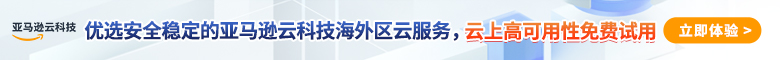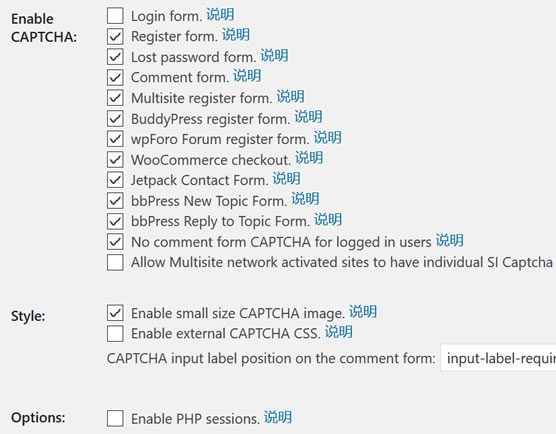# 2种适合WordPress注册验证码插件/非插件实现方法function rand_reg_question(){
\$register_number=rand(0,1); // 设置随机数的返回范围
\$_SESSION['register_number']=\$register_number;
}

global \$register_questions;
// 添加问题数组
// 添加答案数组（与上面的问题对应）

global \$register_questions;
\$register_number=\$_SESSION['register_number'];
?>
<p>
<label><?php echo \$register_questions[\$register_number];?><br />
<input type="text" name="user_proof" id="user_proof" class="input" size="25" tabindex="20" />
</label>
</p>
<?php }

\$register_number=\$_SESSION['register_number'];
if (!isset(\$_POST[ 'user_proof' ]) || empty(\$_POST[ 'user_proof' ])) {
return \$errors->add( 'proofempty', '<strong>错误</strong>: 您还没有回答问题。' );
} elseif ( strtolower( \$_POST[ 'user_proof' ] ) != \$register_answers[\$register_number] ) {
return \$errors->add( 'prooffail', '<strong>错误</strong>: 您的回答不正确。' );
}
}